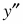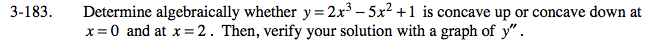### Home > CALC > Chapter 3 > Lesson 3.4.4 > Problem3-183

3-183.

Determine algebraically whether y = 2x3 − 5x2 + 1 is concave up or concave down at x = 0 and at x = 2 . Then, verify your solution with a graph of. Homework Help ✎A function is concave up when its 2nd-derivative is positive and concave down when its 2nd-derivative is negative.

y = 2x³ − 5x² + 1
y ' = 6x² - 10x
y '' = 12x - 10